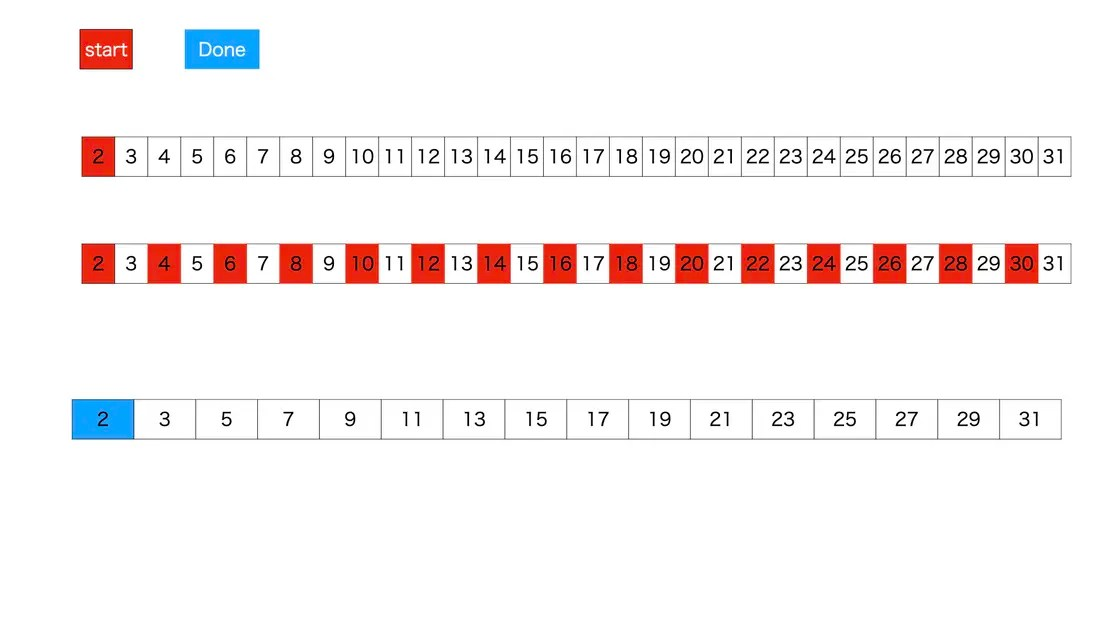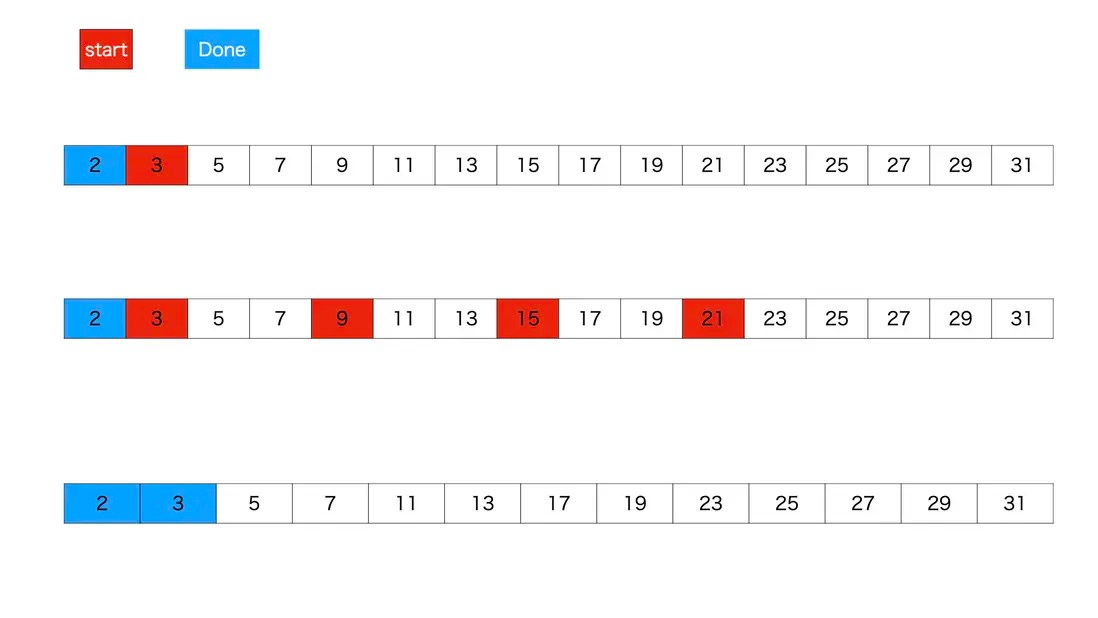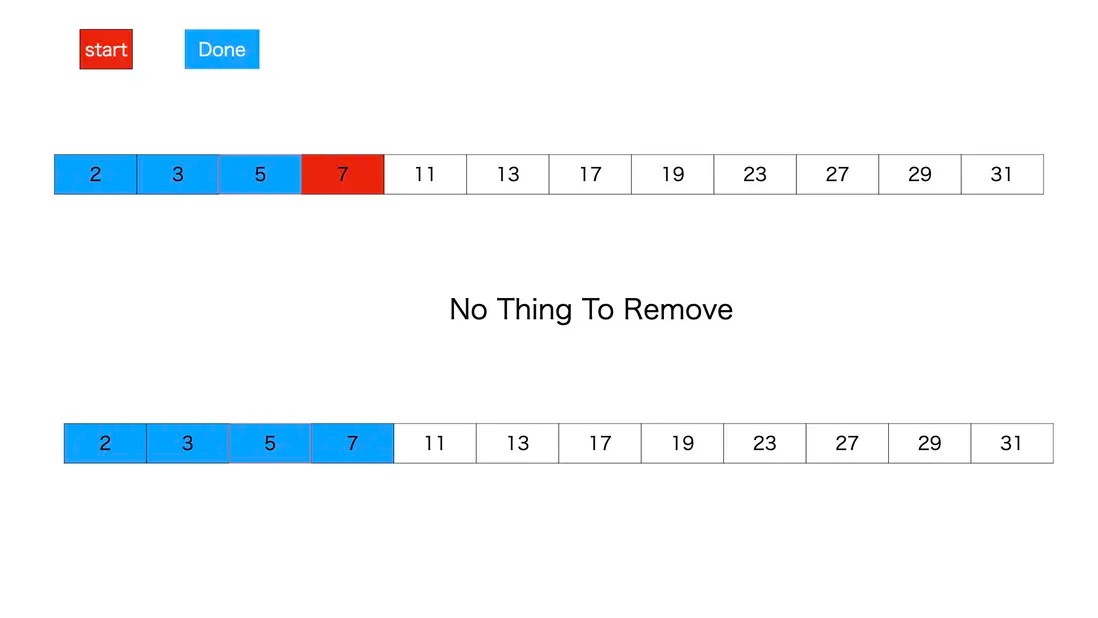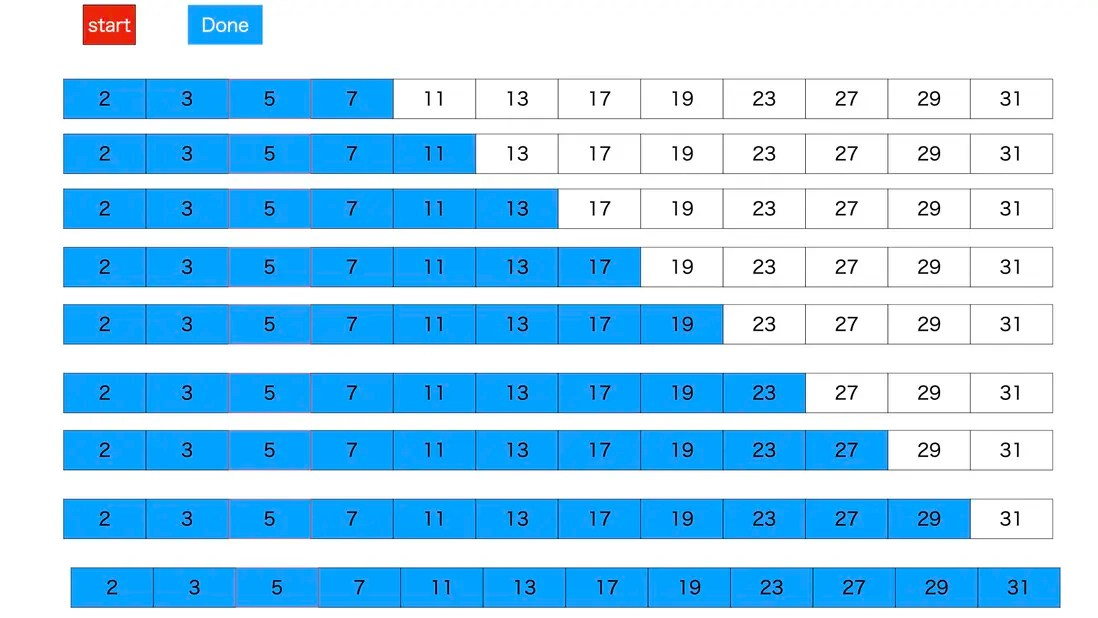# AtCoder ContextABC 084 D - 2017-like Number

Posted Jun 6, 20203 min read

Operation requirements
Run time limit: 2sec
Memory limit: 1024MB
Reprinting without permission is not allowed

Title
If there is an odd number N, N and(N+1//2) are prime numbers, N is a similar number in 2017
Now given Q queries
The i(1<=i<=Q) query, the i-th query gives an odd number li, ri, find the number of x of 2017 similar numbers in the range of li<=x<=ri

Enter prerequisites

• 1<=Q<=100000
• 1<=li<=ri<=100000
• li, ri are odd
• All inputs are integers

Enter
Input according to the following standard input

``````Q
l1 r1
l2 r1
.
.
.
lQ rQ``````

Output
Line i output, the result of the i-th query

example 1
Enter

``````1
3 7``````

Output

``2``

3 and((3+1)/2) are prime numbers, so 3 is the number of similarities in 2017
5 and((5+1)/2) are prime numbers, so 5 is the number of similarities in 2017
7 is a prime number, but 5((7+1)/2) is not a prime number, so 7 is not a similar number in 2017
As mentioned above, the result of query 1 is 2

Example 2
Enter

``````4
13 13
7 11
7 11
2017 2017``````

Output

``````1
0
0
1``````

Note that 2017 is also a similar number of 2017

Example 2
Enter

``````6
1 53
13 91
37 55
19 51
73 91
13 49``````

Output

``````4
4
1
1
1
2``````

Given an interval, among all prime numbers in this interval, select the number that is still prime after being halved

Solving ideas
First we have to see that the maximum value of li and ri is 100000, and the minimum value is 1
li, ri determine the left and right boundaries of the interval
So the maximum span of the interval is 1 to 100,000

First we have to find all the prime numbers in this interval
Because it is a code competition, you cannot use third-party expansion packs
Our handwritten prime generator
The specific method uses the prime screening method
English called sieve of EratosthenesWe take a series of intervals, remember to start at least 2. We find the smallest element of this string of numbers, here 2
First find the multiple of 2 and then remove themFind the first element that has not been traversed from the rest, here is 3
Find multiples of 3, then remove themAnd so on, find multiples of 5 and remove themSometimes, the multiple of the smallest element does not exist, so do nothingIn this way all prime numbers can be found

But one thing to note is the sign of the end of the traversal.
Interval from 2 to the end of the interval
start can traverse from the beginning of the interval to the end of the interval, but if the interval is large, we only need to traverse to the square root of the interval.
For example, 2-16
We only need to traverse to 2-4
2-100 words
We only need to traverse to 2-10

OK, we got all the prime numbers of 1-100000

Let s go through 1-100000 again to determine whether each number is a similar number in 2017
Use the method of cumulative sum to calculate the number of similarities from 1 to traverse point i

Specifically

**If i is the number of similarities in 2017, then
The number of 2017 similar numbers from 1 to i = The number of 2017 similar numbers from 1 to(i-1) + 1**

**If i is not the similarity number of 2017, then
The number of 2017 similar numbers from 1 to i = The number of 2017 similar numbers from 1 to(i-1)**

**Finally, the statistics of the number of 2017 similarities in the interval(li,ri) =
Number of 2017 similarities from 1 to ri-Number of 2017 similarities from 1 to(li-1)**

Code

``````import math

def sushu(n):
arr = []
for i in range(2, n + 1):
arr.append(i)

limit = int(math.sqrt(n)) + 1

prev = []
while True:
start = arr
prev.append(start)
if start> limit:
break
brr = []
for i in arr:
if i%start != 0:
brr.append(i)
arr = brr

prev.extend(arr)
return prev

n = 100000
shushu = sushu(100000)

marks = []
for i in range(n + 1):
marks.append(False)

for j in shushu:
marks[j]= True

marks2 = []
result = []
for i in range(n + 1):
if i%2 == 0:
marks2.append(False)
if i == 0:
result.append(0)
else:
result.append(result[i-1])
else:
if marks[(i + 1) //2]and marks[i]:
marks2.append(True)
result.append(result[i-1]+ 1)
else:
marks2.append(False)
result.append(result[i-1])

Q = int(input())

ARR = []

for i in range(Q):
ARR.append(list(map(int, input().split())))

for i in range(Q):
start = ARR[i]
end = ARR[i]
print(result[end]-result[start-1])``````

to sum up
This question examines the method of seeking prime numbers. Use the prime number screening method to find all prime numbers within a certain interval

Also examines the use of cumulative sums

In addition, I will post some articles on my WeChat personal subscription number, welcome to follow